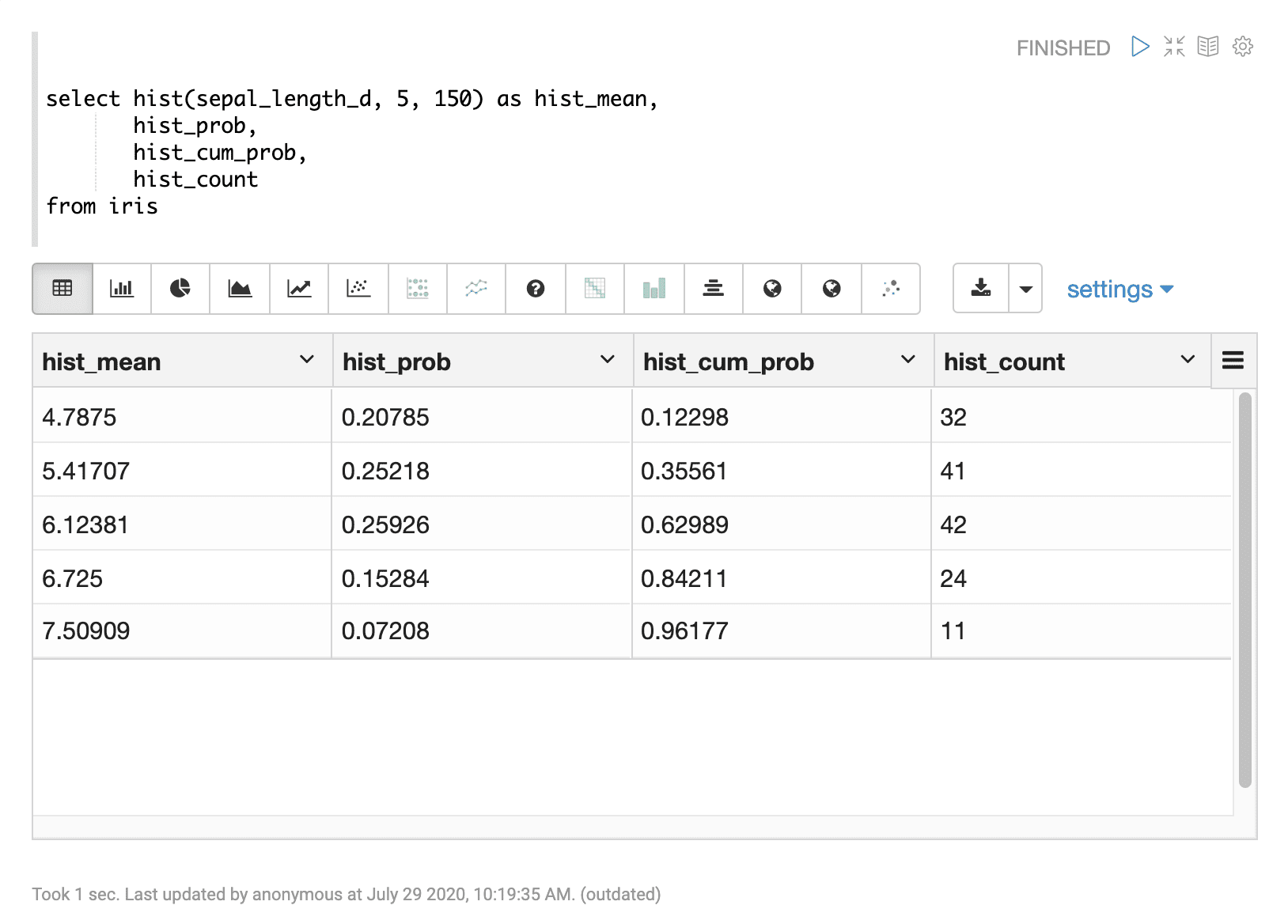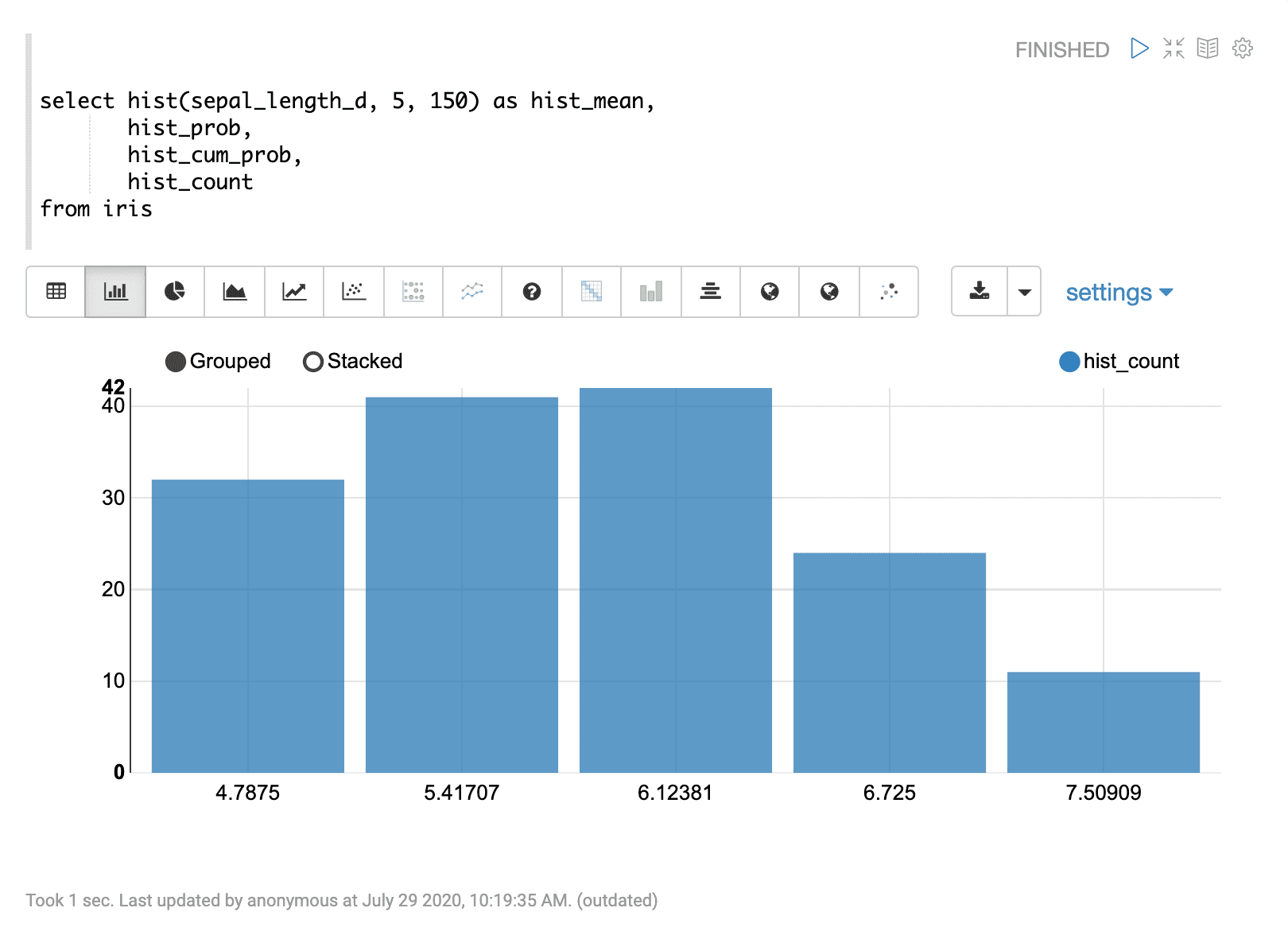# Histogram (hist)

Numeric histograms can be created using the `hist` function. The `hist` function takes three parameters:

1. The numeric field to create the histogram from.

2. The number of bins in the histogram.

3. The sample size to create the histogram from.

## Sample syntax

``````select hist(sepal_length_d, 5, 150) as hist_mean,
hist_prob,
hist_cum_prob,
hist_count
from iris
where species_s = ‘setosa’``````

## Result set

The result set from the histogram will contain one row for each histogram bin. The random sample for the histogram will be drawn from the results that match the WHERE clause in the SQL query. If no WHERE clause is provided the samples will be drawn from the full data set. The `hist` function returns the mean of each bin. There are three additional fields that can be selected when the `hist` function is used:

• `hist_count` : the number of results within each bin.

• `hist_prob`: the probability of the bin, or the percentage of records within each bin.

• `hist_cum_prob`: the cumulative probability of each bin.

Sample result set shown in Apache Zeppelin Table:## Visualization

Histograms can be visualized by plotting the bin means on the x-axis and either the `hist_count`, `hist_prob` or `hist_cum_prob` on the y-axis.

The example below shows a bar chart of the bin means and `hist_count` in Apache Zeppelin: